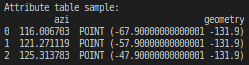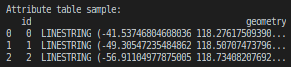# User Guide¶

Below are the parameters that the user should specify.

SUMMARY TABLE
Input Data lon_field_name required only when flatfile
lat_field_name required only when flatfile
azi_field_name required when flatfile or shapefile
angular_error_field_name optional when flatfile or shapefile
Method Parameters k optional
p optional
R optional
runmode 1 : polynomial 2 : distance-weighted
order 0 : global 1 or 2 : local
Output outmode 1 : grid 2 : trajectories

Input Data - Field Names

When input file is a flatfile, all field_names to be used by the program need to be given. When input file is a shapefile, coordinate’s field_names do not need to be given, but the names of the other fields to be used by the program need to be given (e.g. azimuth and azimuth’s angular error). In both cases (either flatfile or shapefile), the user is required to specify the field_name containing azimuth values.

Warning

If azimuth’s angular errors not given, are considered zeros.

Method Parameters - k, p & R

Parameter Explanation
k Polynomial method, degree of the polynomial
p Distance-weighted method, power order for weighted distance
R Distance-weighted method, radius for weighted distance

“ The power values p and the threshold distance R of the weighting effect [Eq. (4)] are important factors. When distance d, increases, the weighting coefficient W i of the datum decreases in a manner depending on the power value p. For a small power value (e.g. p = 1), the weighting coefficient W, decreases slowly with distance; for increasing power values, it decreases more and more rapidly. When p is large ( > 10), the effect will be strong, which indicates that the weighting effects will be concentrated for data with a small distance of d. The disk of radius R represents the smoothing influence field. The smoothing effect takes place in this area only. Generally, one selects a value of R large enough in order to avoid too local effects, because small values of R may result in exaggerated influence of local anomalous data, and also in order to define a reasonable interpolation spatial range. The most significant effects of differences in R values will occur only for relatively small values of p. In fact, the effects of variations of coefficients R and p depend on the local distance effect in a stress field. In practice, one must take into careful consideration (1) the regional geology and tectonic setting, and (2) the geographic distribution of sites and local determination uncertainties. This must be done in each situation in order to select the most appropriate values for the weighting threshold distance R and the weighting power exponent p (see discussion in Lee and Angelier, 1993).” (Lee and Angelier, 1994)

Method Parameters - runmode

Run mode is about which method to use. User can select one of the two methods, entering 1 for Polynomial Method or 2 for Distance-weighted Method. The program has the ability to run both methods if the two options are given seperated with a comma, e.g. 1,2.

Method Parameters - order

For Polynomial Method, coefficient order determines the degree (k) of the polynomial. Specifically, the degree of the polynomial is calculated as k = order + 1 and can reach k = 3, because coefficient order is in [0,2].

For Distance-weighted Method, coefficient order determines the value of the radius (R). Specifically, the degree of distance weight (p) takes the fixed value p = 10 and the value of the radius (R) depends on the extent of the study area and the value of coefficient order.

Warning

When Distance-weighted Method, values 1 and 2 for coefficient order have the same effect.

Output - outmode

Refers to the way that the result will be exported. The program is able to export the result in two different ways, enter 1 for grid map or 2 for trajectories map. The grid map is consists of points, while the trajectories map consists of lines.

It is usefull to define that the trajectories are not equal-value-lines. They indicate the azimuth at each of their point on the plane. So, a trajectories-vector file, does not contain azimuth values. Below are the fields of the attribute table of the corresponding ESRI Shapefiles for each way of display.

Grid TrajectoriesThe result is point geometries. The file contains azimuth value for each grid-node point. The result is linestring geometries, and does not contain azimuth values, as this is indicated by the slope of the vector at each point. Also contains an id number for every trajectory.